PHYSICS

 Time: ONE Hour Max. Marks: 60

• Answers must be written either in English or the medium of instruction of the candidate in high school.
• Answer all the questions in the booklets provided for the purpose.
• There will be no negative marking.
• The relevant working in arriving at an answer has to be shown wherever required. However if the answer can be arrived at without detailed working, write only the answer
• Use of calculators or graph papers is not permitted.
• Questions in part A carry 5 marks each and those in part B carry 2 marks each

Some useful data:

Density of water = 1 g× cm-3

Specific heat of water = 4.2 J g-1K-1=1 cal/g Co

Density of Ice = 0.9 gm/cm3

Latent Heat of Ice = 80 cal/g

PART A

1. On a long journey if 10% of the distance is covered at an average speed of 40 km/h and 90% of the distance is covered at an average speed of 90 km/h. What is the average speed for the entire journey? How does this answer change if the percentages relate to time rather than distance, that is, if 10% of the duration is covered at 40 km/h and 90% of the duration is covered at average speed of 90 km/h.

2. In two glasses there is liquid material of the same type, the volume of one is V1, its temperature of is T1, the volume of the other is V2 and its temperature is T2. We mix the liquids and wait while the temperature settles. What is the common temperature if we neglect any heat losses? What would be the common temperature if the volume of first liquid is halved and the second liquid doubled.
3. Three ice cubes each of side 2 cm at 0° C are dropped into a glass of 18° C water. The volume of the water is 200 cm3. What are the volume and the temperature of the water after the ice cubes have melted?

4. How much can the temperature of the water in a 50 m x 25 m x 2 m size pool be raised with the energy released by a single gram of 235 Uranium isotope in a nuclear power plant? Assume 47% of the energy (200 MeV of energy is liberated in the fission of one isotope) is used to heat up the water.

5. Two cars are 60 km away from each other and are moving towards each other at 116 km/h and 184 km/h. How far are they from each other one and a half minutes before their collision?

 A ray of light is incident on an isosceles right-angled prism made of a material of critical angle 42° . Find the distance of point of emergence of the ray from the prism if AB = d and AP = L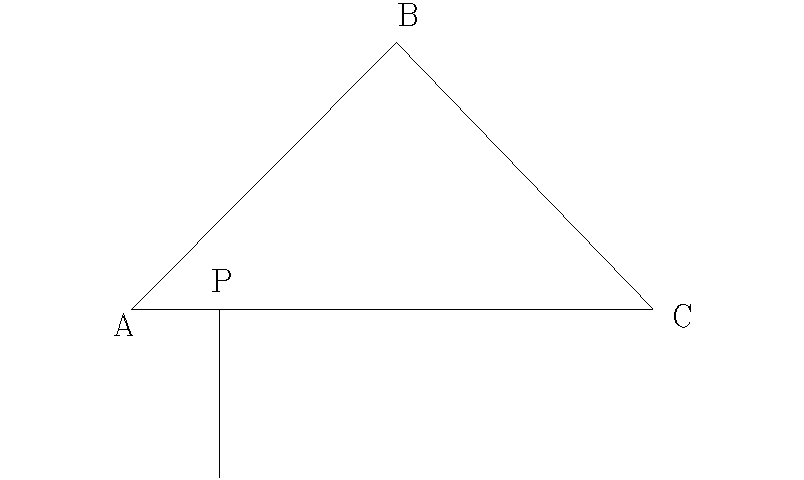Large number of identical blocks are placed on a surface with adjacent blocks d apart. The left most block is imparted a velocity V to the right. On striking its neighbour it comes to rest and the struck block takes off at 0.8 V. This process repeats. What is the time interval between the third and fourth hits? Assume that the motion between the hits is uniform. Neglect the duration of collision.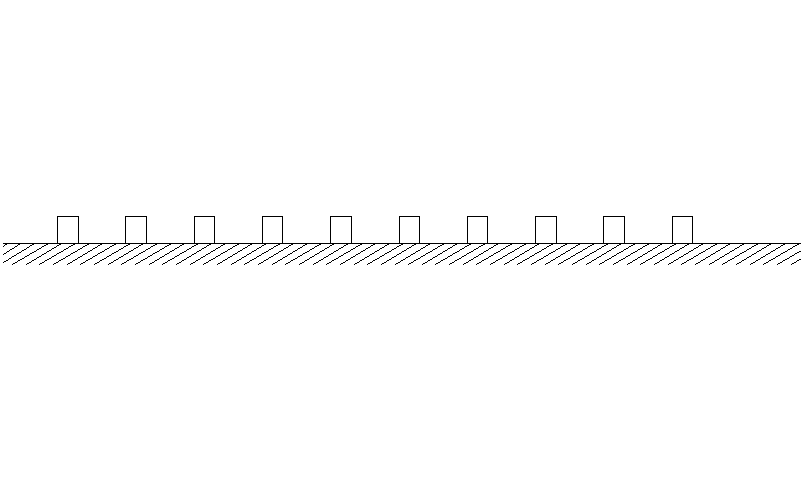A stick of height h is protruding out of a liquid contained in a large vessel with half of it inside the liquid. Rays of light from after noon sun are incident on the stick at 45° as shown by the dotted lines. Find the length of the shadow on the bottom of the vessel. Would the shadow length increase or decrease as the sun goes down further?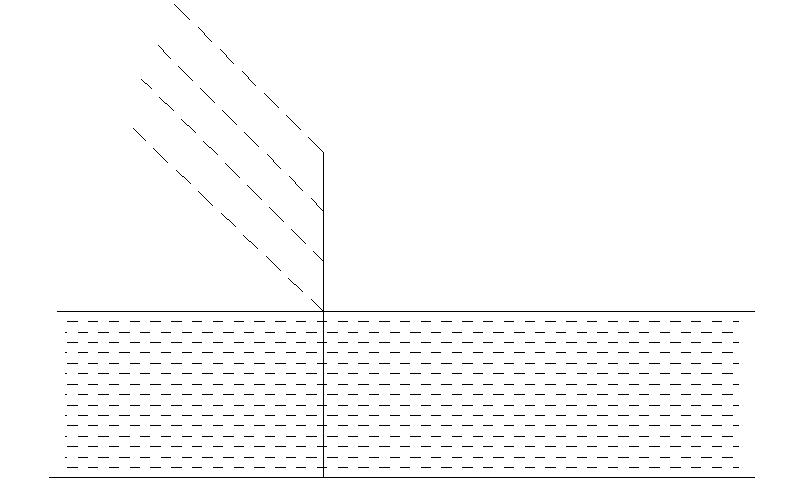The wire mesh shown is made of a uniform wire. Resistance between any two points is R. When wires OH and OD are removed some current flows between the terminals when a potential difference V is applied between them. If now the wires OB and OF are also removed, find the change in potential difference for same current to flow between the terminals.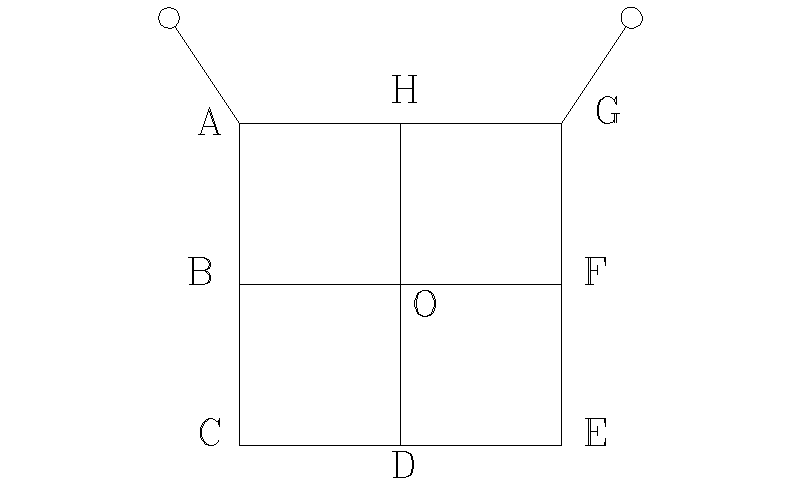1. Ratio of specific heats ‘g ’ of a mixture of gases is given by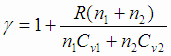where Cv1 and Cv2 are the specific heats at constant volume of the two gases in the mixture and g is the ratio of specific heats, n1 and n2 are the number of moles of the gas in the given mixture.

Find the velocity of sound in a mixture by equal volumes Hydrogen and Helium at 27° C. Specific heats at constant volume for Hydrogen and Helium are 5R/2 and 3R/2 respectively.

PART B

1. Two pendulums are drawn aside and released at the same time. One has a time period of 2 s and the other 2.5 s. What is the least time when they are at their extreme positions on opposite sides of equilibrium?

2. At a given time, the distance recorder of a car cruising at near constant speed on a road showed 24, 942 km. Two hours later the driver noticed that it showed a symmetric number again. What was the average speed of the car in this two-hour leg?

3. Explain why a glass of water quickly warms up when placed on a hot surface but does not cool as quickly when placed on a cold surface. Assume same temperature differences between the water and the surfaces in both the cases.

4. Two resistances, a 60 ohm and an unknown one are connected to a power source of 120 V in a series arrangement. This way the power of the unknown resistance is 60 W. What is the Unknown resistance?

5. A hollow sphere made of material of density 3 g/cm3 is floating with three fourth of its volume submerged in a liquid of density 0.8 g/cm3. If the external volume of the sphere is 200 cm3, find the volume of the cavity inside the sphere.